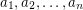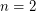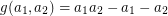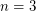Importance: Medium ✭✭
 Author(s):
 Subject: Number Theory » Combinatorial Number Theory
 Keywords:
 Posted by: maxal on: November 26th, 2008
Problem   Find an explicit formula for Frobenius numberof co-prime positive integersfor.

For, the formulawas discovered by Sylvester discovered in 1884 [S]. For, an explicit solution is also known [G,R,SB]. No explicit solution is known for.

## Bibliography

[G] Greenberg, H. "Solution to a Linear Diophantine Equation for Nonnegative Integers." J. Algorithms 9, 343-353, 1988.

[R] Rødseth, Ø. J. "On a Linear Diophantine Problem of Frobenius." J. reine angew. Math. 301, 171-178, 1978.

[SB] Selmer, E. S. and Beyer, Ö. "On the Linear Diophantine Problem of Frobenius in Three Variables." J. reine angew. Math. 301, 161-170, 1978.

[S] Sylvester, J. J. "Question 7382." Mathematical Questions from the Educational Times 41, 21, 1884.

* indicates original appearance(s) of problem.